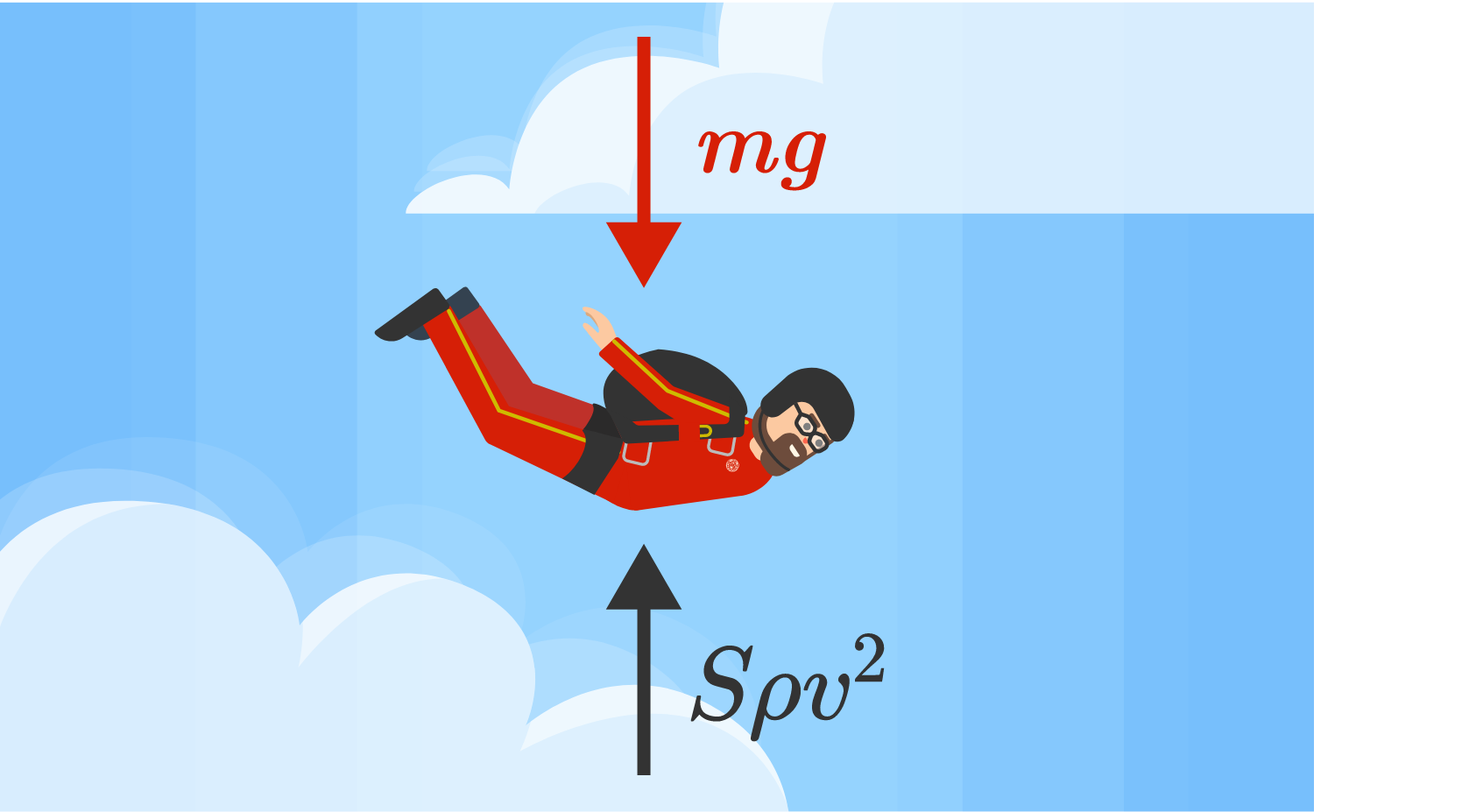## How High Can You Dive From?

In an attempt to set a world-record high dive, High Divin' Hidalgo wants to dive into a pool from a diving board in the clouds. To pull off this stunt, the pool will be extra deep and air will be bubbled into the pool to soften his impact with the water. Will he survive this breathtaking leap?

On Earth, we feel the force of Earth's gravity every day. When there's no floor or ground underneath you to push back, perhaps because you just jumped out of an airplane, you accelerate towards the Earth at a constant rate of roughly $$\SI[per-mode=symbol]{10}{\meter\per\second\squared},$$ often referred to as the gravitational acceleration $$g.$$If you were to jump out of a plane, you wouldn't accelerate at $$\SI[per-mode=symbol]{10}{\meter\per\second\squared}$$ all the way down. As your speed increases, the air you're falling through pushes back on you. This upward drag force reduces your acceleration, and can be calculated by:

$F_\textrm{D} = S\rho v^2$

where $$S$$ is your "effective" surface area (which depends both on how big your area is and how aerodynamically you are shaped), $$\rho$$ is the density of the fluid you're moving through, and $$v$$ is your velocity.If you fall for a long enough time, your speed will be high enough that the drag force is equal and opposite to the force of Earth's gravity (which is your mass times $$g$$). At this speed, known as terminal velocity, the net force on you is zero and you stop accelerating:

$F_\textrm{net} = S\rho_\textrm{air}v_\textrm{terminal}^2 - mg = 0$

Someone falling at terminal velocity has a net force of zero on them, so their speed will only change if the balance between gravity and drag force on them changes. A skydiver releasing their parachute, for example, will increase the $$S$$ term in the drag force equation, so their drag force will become greater than their gravitational force and they'll begin to slow down.

Similarly, if a someone were to fall into water at terminal velocity, then the $$S$$ and $$v$$ terms in the drag force equation would both remain the same. However, $$\rho$$ would increase from roughly $$\SI[per-mode=symbol]{1.2}{\kilo\gram\per\meter\cubed}$$ for air to $$\SI[per-mode=symbol]{1000}{\kilo\gram\per\meter\cubed}$$ for water, causing the drag force to increase sharply by a factor of almost $$1000.$$

This sudden increase would be catastrophic for High Divin' Hidalgo, and is why falling into water at very high speeds isn't any safer than falling onto the ground at high speed: in both cases your body will experience extremely high accelerations upon impact. However, our friend Hidalgo's hopes rest on reducing the density of the pool water with bubbles, which in turn reduces the acceleration he experiences as his body breaks the water's surface.

# Today's Challenge

Hidalgo dives off the high board and reaches his terminal velocity before hitting the pool's surface.If the highest instantaneous acceleration that Hidalgo can withstand is $$100g,$$ what is the required volume fraction of bubbles at the surface of the pool such that he can complete the dive safely?

Hint: Hidalgo's acceleration is $$a=F_\textrm{net}/m,$$ where $$m$$ is his mass.

×

Problem Loading...

Note Loading...

Set Loading...